# SR Flip Flop or SR Latch: What is it? (Plus Truth Table)

Contents

## What is an SR Flip Flop?

An SR Flip Flop (also referred to as an SR Latch) is the most simple type of flip flop. It has two inputs S and R and two outputs Q and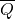. The state of this latch is determined by the condition of Q. If Q is 1 the latch is said to be SET and if Q is 0 the latch is said to be RESET. This SR Latch or Flip flop can be designed either by two cross-coupled NAND gates or two-cross coupled NOR gates.

When we design this latch by using NOR gates, it will be an active high S-R latch. That means it is SET when S = 1. When we design this latch by using NAND gates, it will be an active low S-R latch. That means it is SET when S = 0. SR Flip Flop is also called SET RESET Flip Flop.

The figure below shows the logic circuit of an SR latch.

In the above logic circuit if S = 1 and R = 0, Q becomes 1. Let us explain how.

• NOR gate always gives output 0 when at least one of the inputs is 1.
• So when S is applied as 1 the output of gate G2 i.e.is 0 irrespective of the condition of second input Q to the gate.
• Nowis the input of gate G1 so both the inputs of G1 become 0 as R is already 0. So, the output of G1 is now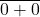or 1.
• So whatever may be the previous condition of Q, it always becomes Q = 1 and= 0 when S = 1 and R = 0. This is called the SET condition of the latch.

In the above logic circuit if S = 0 and R = 1, Q becomes 0. Let us explain how.

• As we already said, a NOR gate always gives output 0 when at least one of the inputs is 1.
• So when R is applied as 1, the output of gate G1 i.e. Q is 0 irrespective of the condition of the second inputto the gate.
• So, whatever may be the previous condition of Q, it always becomes 0 this 0 is then fed back to the input of gate G2. As here S is already 0, both inputs of G2 are 0. Hence the output of G2 i.e.will be 1. So, Q = 0 and= 1 when, S = 0 and R = 1. This is called the RESET condition of the latch.

In the above logic circuit if S = 0 and also R = 0, Q remains the same as it was. Let us explain how.

• First suppose Q is previously 1.
• Now the inputs of G2 are 0 and 1 as S=0 and Q=1. So output of G2 i.e.is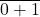or 0.
• Now both inputs of G1 are 0 as R=0 and=0. So the output of G1 i.e. Q is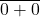or 1.
• Now suppose Q is previously 0.
• Now both inputs of G2 are 0 and 1 as S = 0 and Q = 0. So the output of G2 i.e.isor 1.
• Now the inputs of G1 are 0 and 1 as R=0 and= 1. So the output of G1 i.e. Q is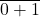or 0.
• So it is proved that Q remains the same as it is when S = 0 and also R = 0 in SR latch or flip flop.

In the above logic circuit if S = 1 and also R = 1, the condition of Q is totally unpredictable. Let us explain how.

• First suppose Q is previously 1.
• Now both inputs of G2 are 1 as S = 1 and Q = 1. So output of G2 i.e.is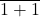or 0.
• Now the inputs of G1 are 1 and 0 as R = 1 and= 0. So the output of G1 i.e. Q is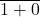or 0. That means Q is changed.
• Now Q is 0. So inputs of G2 are 1 and 0 as S = 1 and Q = 0. So the output of G2 i.e.isor 0. That meansis unchanged.
• Now the inputs of G1 are 1 and 0 as R = 1 and= 0. So the output of G1 i.e. Q isor 0. That means Q is unchanged.

So, when both S and R are 1, it becomes unpredictable whether the value of output Q will be changed or unchanged. This condition of SR latch normally avoided. As the latch is SET when S = 1(HIGH), the latch is called Active High SR Latch.

There is another type of latch which is SET when, S = 0 (LOW), and this latch is known as Active Low SR Latch. There are also D Flip Flops, JK Flip Flops, SR Flip Flops, Clocked SR Flip Flops.

## SR Flip Flop Truth Table

The truth table for an SR Flip Flip (i.e. SR Latch) has been shown in the table below.

You can learn more about SR flip flops and other logic gates by checking out our full list of logic gates questions.

Want To Learn Faster? 🎓
Get electrical articles delivered to your inbox every week.
No credit card required—it’s 100% free.